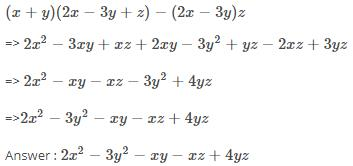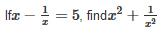Courses

# Test: Algebraic Expressions And Identities- 3

## 20 Questions MCQ Test Mathematics (Maths) Class 8 | Test: Algebraic Expressions And Identities- 3

Description
This mock test of Test: Algebraic Expressions And Identities- 3 for Class 8 helps you for every Class 8 entrance exam. This contains 20 Multiple Choice Questions for Class 8 Test: Algebraic Expressions And Identities- 3 (mcq) to study with solutions a complete question bank. The solved questions answers in this Test: Algebraic Expressions And Identities- 3 quiz give you a good mix of easy questions and tough questions. Class 8 students definitely take this Test: Algebraic Expressions And Identities- 3 exercise for a better result in the exam. You can find other Test: Algebraic Expressions And Identities- 3 extra questions, long questions & short questions for Class 8 on EduRev as well by searching above.
QUESTION: 1

### Which of the following is an expression?

Solution: (c) Becoz highest power of variable 'x' is not equal to zero . ..
QUESTION: 2

### Which of the following is a binomial?

Solution:

An algebraic expression consisting of unlike terms is called a binomial.

so, 2x+7 is a binomial as it contains two unlike terms i.e 2x and 7.

QUESTION: 3

### Expressions consists of _____________ and _______________.

Solution: Variables and constants .This is because an expression is made up of these only.
QUESTION: 4

What degree does x3 - x2y2 - 8y2+ 2 have?

Solution:

Monomial means expression containing only one term and option B has one term so it is a monomial.

QUESTION: 5

When numbers/literals are added or subtracted, they are called _________.

Solution:
QUESTION: 6

Multiply (a + 7) and (b – 5).

Solution:

(a+7)(b-5) = a(b-5)+7(b-5) = ab-5a+7b-35

QUESTION: 7

Simplify: (x + y) (2x – 3y + z) – (2x – 3y)z

Solution:QUESTION: 8

If (x+1/x) = 6, find  x2+1/x2

Solution: X²+1/x² can be written as (x+1/x)²-2 on putting values we have 6²-2=34
QUESTION: 9Solution:
QUESTION: 10

Which of the following is an expression?

Solution: Option C is correct bcuz, an expression consists of at least one variable like :- x, y, z as well as a number. An expression can also be like this :- 2xy, 3y², 5xy+8, 6z²-3, etc
QUESTION: 11

Which of the following is a trinomial?

Solution: Because monomial 1 term binomial, 2 terms and trinomial 3 terms therefor 7_3x+4y trinomial.
QUESTION: 12

Which of the following is like term as 3xy2?

Solution:
QUESTION: 13

Subtract 3x (x – 4y + 5z) from 4x (2x – 3y + 10z).

Solution:
QUESTION: 14

n (4 + m) = 4n + ___

Solution: N(4+m) = 4n + ___Here , n is multiplied by 4 which is added to n multiplied by m.so, n×4= 4n n×m= nm so the answer is 4n + nm.
QUESTION: 15

Using identity evaluate 297 × 303.

Solution:
297×303
(300-3)(300+3)
(300)whole square (3)whole square
90000-9
89991. identity
{a-b} {a+b} ={a square} - {b square}
QUESTION: 16

Simplify: (1.5x – 4y)(1.5x + 4y +3) – 4.5x +12y

Solution:
(1.5x - 4y) (1.5x + 4y + 3) - 4.5x + 12y
= 1.5x(1.5x + 4y +3) - 4y(1.5x + 4y +3) - 4.5x +12y
= (1.5x X 1.5x) + (1.5x X 4y) + (1.5x X 3) - (4y X 1.5x) - (4y X 4y) - (4y x 3) - 4.5x +12y

= 2.25x^2 + 6xy + 4.5x - 6xy - 16y^2 - 12y - 4.5x + 12y
= 2.25x^2 + ( 6 - 6)xy + (4.5 - 4.5)x - 16y^2 + (12 - 12)y
= 2.25x^2  + 0 + 0 - 16y^2 + 0
= 2.25x^2 - 16y^2
QUESTION: 17

If x + y = 12 and xy = 32, find the value of x2+ y2.

Solution:
x=8 or 4 and y=4 or 8 so,x^2+y^2=64 +16 or 16 +64 =80 and that is option A.
QUESTION: 18

(x – a) (x + a) = ?

Solution: (a-b)(a+b) = a^2 - b^2(x-a)(x+a) = x (x+a) -a (x+a) = x^2 + ax -ax -a^2 = x^2 - a ^2
QUESTION: 19

Which of the following is a trinomial?

Solution:
QUESTION: 20

If x = 3 is solution of x² + kx + 15, value of k is

Solution:

Since solution is x=3, this means the equation is equal to 0 when x=3, substituting the values gives k=-8